# Long Division - Introduction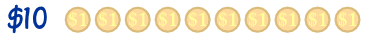John and Ann are given \$10 to share. How do they share it? Easy! \$5 each.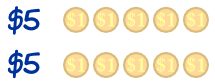But then they think of their little baby brother Max.

"Maybe we should share it with him?" they ask each other.

So how much do they each get?

\$10 shared amongst 3 people

That is \$3 each ... but 3 lots of \$3 is \$9: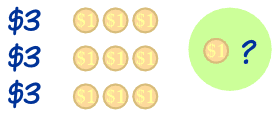That leaves \$1 still to share.

Let us break that \$1 into ten 10c pieces: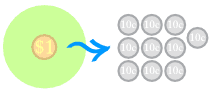OK. Let's share those 10 cent pieces. That is an extra 30 cents each: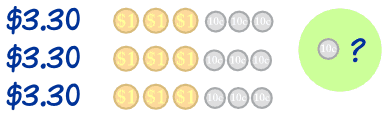But that still leaves 10c !!!

So let us turn the 10c into ten 1c pieces: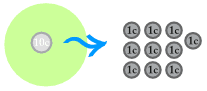OK, share that too: they each get 3c more: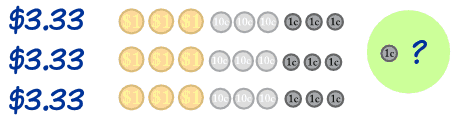That leaves one cent! But we can't break that cent any further so it
is simply "left over", which we call the "remainder"

The answer is: they each get \$3 and another 30c and another 3c for a totsl of \$3.33 each, with one cent left over!

\$3.33 each (with a remainder of 1c)

And ...

That is how Long Division works!

In Long Division we:

• do the best division we can,
• then find out what is left over, and try to divide that,
• around and around until we can't go any further!

## It is Written Down in a Special Way

First, we write down that we want to divide \$10 by 3 like this:Note: We don't use the \$ symbol, instead we write
the \$10 as the number 10.00 meaning 10 dollars and 0 cents

Then we write down that we took 3 lots of \$3 to make \$9:We write the 9 below the 10, because the next thing to do is to subtract \$9 from \$10 to find we still have \$1 left to divide:Next is to repeat the whole thing, but do it for the \$1 (which is written as 1.00):That leaves 10 cents, or 0.10 yet to divide, so we repeat again:We can't divide any more, so that is our answer!

\$10 divided by 3 is \$3.33 with \$0.01 (1 cent) remainder

Now have a look at this Long Division Animation

652,1985,1986